##### Geometry: 1,001 Practice Problems For Dummies (+ Free Online Practice)A rhombus is a parallelogram with some interesting and useful properties. For example, all of its sides are congruent, and it contains diagonals that are perpendicular bisectors and that bisect the angles of the rhombus.

You can use these properties in the following practice geometry questions, first, to solve for a missing variable x, and second, to find the perimeter of a rhombus.

## Practice questions

1. In rhombus LMNO, diagonals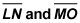intersect at P. If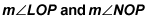are represented by 3x and 2x + 25, respectively, find the value of x.

2. In rhombus DEFG, diagonals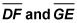intersect at R. If GR = 3 and DR = 4, find the perimeter of rhombus DEFG.

1. 25

The diagonals of a rhombus bisect the angles of the rhombus, and a bisector divides an angle into two congruent angles. Therefore,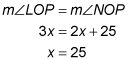2. 20

The diagonals of a rhombus are perpendicular bisectors, which means they form right angles at their point of intersection.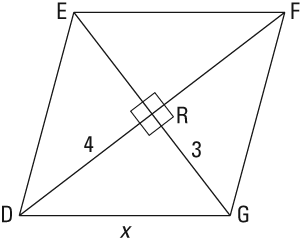This creates four right triangles within the rhombus. Using the Pythagorean theorem to find the hypotenuse of one of the right triangles will give you the length of one of the sides of the rhombus: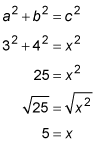All four sides of a rhombus are congruent, so in this case, each of the sides of the rhombus is equal to 5. The perimeter of a rhombus is equal to the sum of the four sides of the rhombus: 5 + 5 + 5 + 5 = 20.